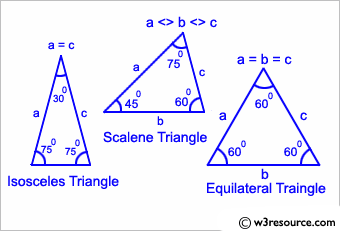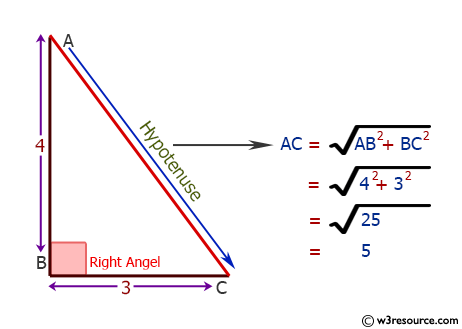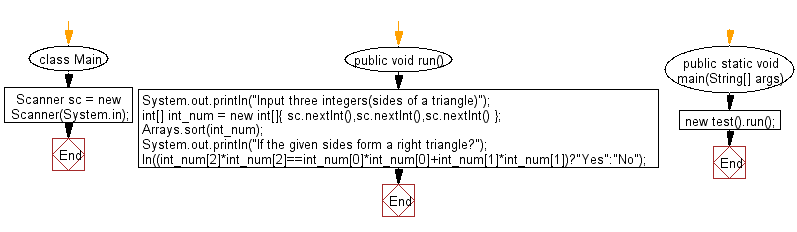# Java Exercises: Check whether three given lengths of three sides form a right triangle

## Java Basic: Exercise-213 with Solution

Write a Java program to check whether three given lengths (integers) of three sides form a right triangle. Print "Yes" if the given sides form a right triangle otherwise print "No".

Input:

Integers separated by a single space. 1 ≤ length of the side ≤ 1,000

Pictorial Presentation:Sample Solution:

Java Code:

``````import java.util.Arrays;
import java.util.Comparator;
import java.util.Scanner;
class Main {
Scanner sc = new Scanner(System.in);
public void run() {
System.out.println("Input three integers(sides of a triangle)");
int[] int_num = new int[]{
sc.nextInt(),sc.nextInt(),sc.nextInt()
};
Arrays.sort(int_num);
System.out.println("If the given sides form a right triangle?");
ln((int_num*int_num==int_num*int_num+int_num*int_num)?"Yes":"No");
}
public static void main(String[] args) {
new Main().run();
}
public static void pr(Object o) {
System.out.print(o);
}
public static void ln(Object o) {
System.out.println(o);
}
public static void ln() {
System.out.println();
}
}
```
```

Sample Output:

```Input three integers(sides of a triangle)
6 9 12
If the given sides form a right triangle?
No
```

Flowchart:Java Code Editor:

What is the difficulty level of this exercise?

﻿

## Java: Tips of the Day

Parsing dates:

```import java.io.*;
import java.util.*;
import java.text.*;

String s = "2001/09/23 14:39";

SimpleDateFormat formatter = new SimpleDateFormat ("yyyy/MM/dd H:mm");
Date d = formatter.parse(s, new ParsePosition(0));
```